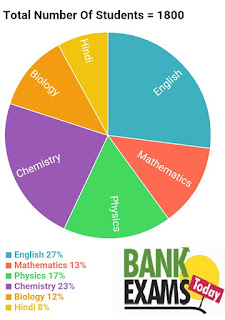New Students Offer - Use Code HELLO

# Data Interpretation Quiz - 2 for IBPS Clerical Pre Exam 2016

### Study the following Pie-chart carefully and Answer the questions given below :

In school total number of students passed in different subjects were as per given pie-chart .Click Image to Zoom
1. If students passed in Chemistry increased 26 and Physics students decreased 26. Find the students passed in Physics approximately what percentage in Chemistry ?
1.64%
2.72%
3.76%
4.56%
5.68%

2. What is the difference between the total number of students who passed in English and Physics together and the total number of students passed in Hindi , Biology and Chemistry . After few days 20 students from English and 35 students from Biology declared failed due to malpractice in exams ?

1.36
2.72
3.33
4.56
5.42

3. Find ratio between students passed in English and Chemistry , If students passed in Chemistry Increased 69 ?

1. 81:63
2. 91:101
3. 81:85
4. 14 : 27
5. 11:24

4. If the percentage of Mathematics students passed in Exam is increased by 50% and percentage of Students passed in Hindi is decreased by 25%  , then what will be the total number of students passed in Mathematics and Hindi together?

1. 406
2. 459
3. 457
4. 471
5. 432

5. If 2/9 th of students who passed in Physics are female , then number of male Students passed in  Physics is approximately what per cent of total number of students passed in Chemistry?

1. 58
2. 61
3. 73
4. 53
5. 51

1.1

Chemistry = 18*23 = 414
After increasing 26 students = 414+26 =440

Physics = 17*18 = 306
After decreasing 26 students = 306-26=280

percentage = 280*100/440= 63.63~= 64%

2.3

English  = (27*18)-20 =486-20 =466
Physics = 17*18 = 306

Hindi = 8*18 =414
Chemistry = 18*23 = 414
Biology = 12*18 -35= 216-35= 181

Difference = ( English + Physics) - ( Hindi + Chemistry + Biology  )

==> difference = 772-739 = 33

3.1

Students passed in English = 18*27 =486

Students passed in Chemistry =18*23=414

after increasing 69 in Chemistry = 441+69= 510

Ratio between English and Chemistry = 486 : 510 =81:85

4.2

Total together = 1800 ( 13*150/(100*100) + 8*75/(100*100))

==> Total together = 351+108 = 459

5. 1

Male students passed in Physics = 7/9 * 1800 * 17/100 = 238
Students passed in Chemistry = 23*1800/100 = 414

percentage = 238/414 *100 = 57.48